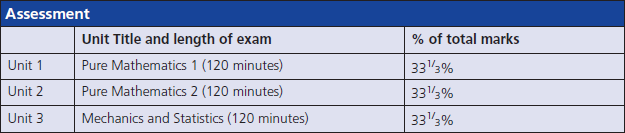# Further Mathematics

EXAMINATION BOARD: Edexcel

As well as being a highly regarded subject in its own right, Mathematics is an excellent partner for many other subjects, especially Sciences, Geography and Economics. At A Level, Mathematics is divided into two branches, Pure Maths (the Core units) and Applied Maths (Mechanics and Statistics). In Pure Maths pupils will develop and extend skills in algebra and trigonometry, as well as meeting new topics such as differentiation and integration (calculus), logarithms, functions, vectors and mathematical proof. Mechanics looks at how algebraic techniques can be used to model real-world problems, with a particular emphasis on Newton’s Laws (which pupils may already have met in Physics)  applied to systems of forces. In Statistics pupils will use mathematical techniques to analyse and interpret data, and see how mathematical probability models such as the normal distribution can be used to predict future outcomes, assessing the reliability predictions using the concept of hypothesis testing; this will include the use of technology to explore large sets of real world data.

Pupils will:

• Learn how to analyse and solve increasingly complex problems, developing the use of logic in mathematical arguments.
• Appreciate the importance of rigorous proof in establishing mathematical concepts.
• Apply algebraic methods to model the physical world.
• Develop the ability to make inferences and predictions based on detailed analysis of data.

## course specification